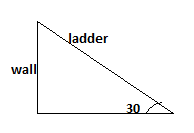0
387

# Time And Distance Questions For LIC AAO

Download Important Time and Distance questions for LIC AAO exam. Top 10  Time and Distance questions with answers based on previous year asked questions.

Question 1: If a student walks from his house to school at 5 km/hr, he is late by 30 minutes. However, if he walks at 6 km/hr, he is late by 5 minutes only. The distance of his school from his house is.

a) 2.5 km

b) 3.6 km

c) 5.5 km

d) 12.5 km

Question 2: An express train travelled at an average speed of 100km/hr, stopping for 3 minutes after 75 km. A local train travelled at speed of 50 km/hr, stopping for 1 minute after every 25 km. If the train began travelling at the same time, how many kms did the local train level in the time it took the express train to travel 600 km ?

a) 307.5 km

b) 900 km

c) 1000 km

d) 1200 km

Question 3: A 10 metre, long ladder is placed against a wall. It is inclined at angle of 30C to the ground. The distance of the foot of the ladder from the wall is

a) 7.32 m

b) 8.26 m

c) 8.66 m

d) 8.16 m

Question 4: A squirrel starts climbing up a tree at the speed of 6 metres per minute but after each 6 metre it slips down 4 metres. It will be able to reach a top of 120 metre high in:

a) 20 minutes

b) 60 minutes

c) 58 minutes

d) $1\frac{1}{2}$ hours

Question 5: Two trains running in opposite directions cross a man standing on the platform in 27 seconds and 17 seconds respectively and they cross each other in 23 seconds. The ratio of the their speed is:

a) 1: 3

b) 3: 2

c) 3: 4

d) None of these

Question 6: A plane left 30 minutes later than the scheduled time and in order to reach the destination 1500 km away in time, it had to increase the speed by 250 km/hr from the usual speed. Its usual speed is:

a) 720 km/hr

b) 730 km/hr

c) 740 km/hr

d) 750 km/hr

Question 7: A 153 metre long train is running at 144 kmph. What time will it take to cross a 207 metre long platform ?

a) 11 seconds

b) 10 seconds

c) 8 seconds

d) 9 seconds

e) None of these

Question 8: A boatman rows upstream at the rate of 13 kmph and downstream at the rate of 20 kmph for the same distance. Find the rate of boat in still water.

a) 16 kmph

b) 16.5 kmph

c) 17 kmph

d) 17.5 kmph

e) None of these

Question 9: There are 77 equidistant trees on each side of a 1444 metre long road. Find the distance between the two consecutive trees.

a) 19 metre

b) 18 metre

c) 18.75 metre

d) 17 metre

e) None of these

Question 10: Ram takes 30 minutes to reach office from home by car. One day, due to heavy traffic he drove his car at (4/5)th of original speed for (1/3)rd of total distance and remaining distance at (2/5)th of the original speed. Find out the time taken by Ram on that day?

a) 60 minutes

b) 75 minutes

c) 65 minutes

d) 67.5 minutes

e) 62.5 minutes

The express train will cover 600 km in 6hrs and will stop fo 21 minutes in total at different stops.
Now, the distance covered by the local train in 6 hours 21 minutes while subtracting the 1 minute for each halt after 25 minutes = 6.35 * 50 – 250/25 = 307.5 km.

Therfore, the correct option is option A.

This can be represented in diagrammatic form as,Hence, by 30-60-90 theorem
Side opposite to 60 degree angle = $\sqrt{3}$/2 *hypotenuse = $\sqrt{3}$/2 *10 = 8.66 m

Therefore, the correct option is option C.

The aggregate distance covered by squirrel in 1 minute = 6-4 = 2 metres.
So, at the end of 1 minute squirrel will climb 2 metres, at the end of 2 minutes squirrel will climb 4 metres and so on.
At the end of the 57 minute squirrel will have climbed 114 metres.
Now, at the 58th minute it will advance 6 metres more and reach the top.
Therefore, the  correct option is option C.

Let the lengths of train be x and y.
Now their speed is (x/27) and (y/17)
Also, they cross each other in 23 seconds.
(x/27) + (y/17) = (x+y)/23
We get (4/621)x = (6/291)y
(x/27)*(4/23) = (102/291)*(y/17)
(x/27)/(y/17) = (102/291)*(23/4)
Ratio of speed = (391/194)
Option D is the correct answer.

The plane left half an hour late i.e it had to cover the same distance in half hour less time.
Now, we know that time = distance/speed
Let the origibal speed of the plane be x.
Now, (1500/x – 1500/x+250) = 0.5
Solving we get x = 750 km/hr.
Hence, the correct answer is option D.

Then his original speed = $\frac{150d}{30}$ = 5d
Distance that Ram covered at (1/3)rd of original distance = $\frac{150d}{3}$ = 50d
(4/5)th of original speed = $\frac{4}{5} * 5d$ = 4d
Time taken by Ram to cover this distance = $\frac{50d}{4d} = 12.5$minutes
(2/5)th of original speed = $\frac{2}{5}*5d$ = 2d
Time taken by Ram to cover the remaining distance = $\frac{100d}{2d}$ = 50 minutes